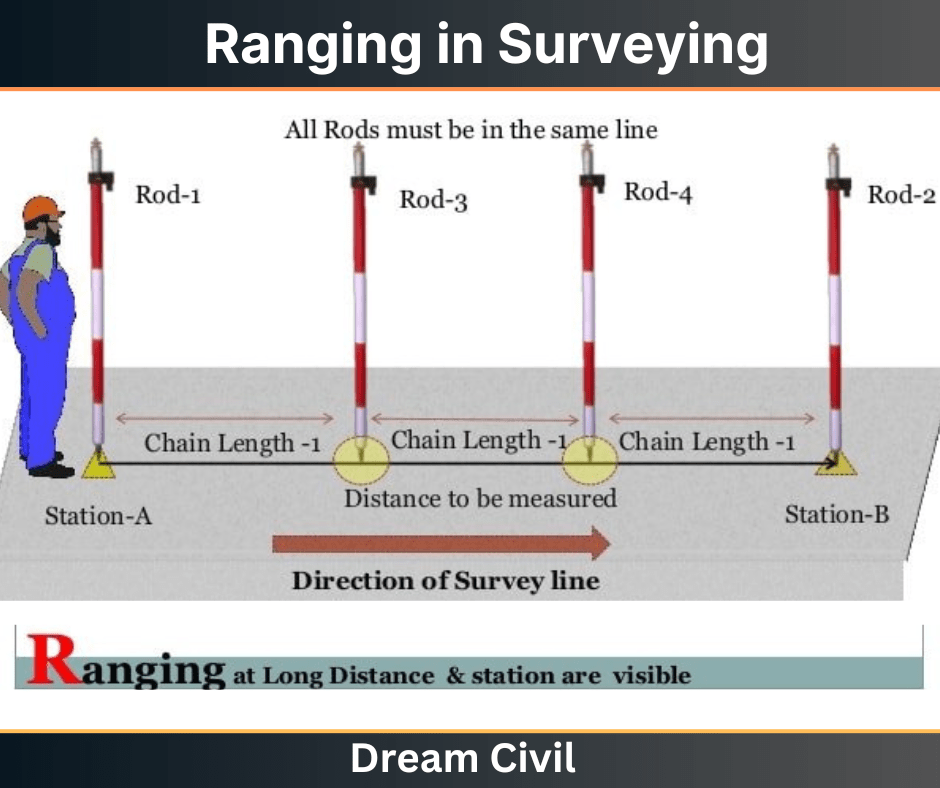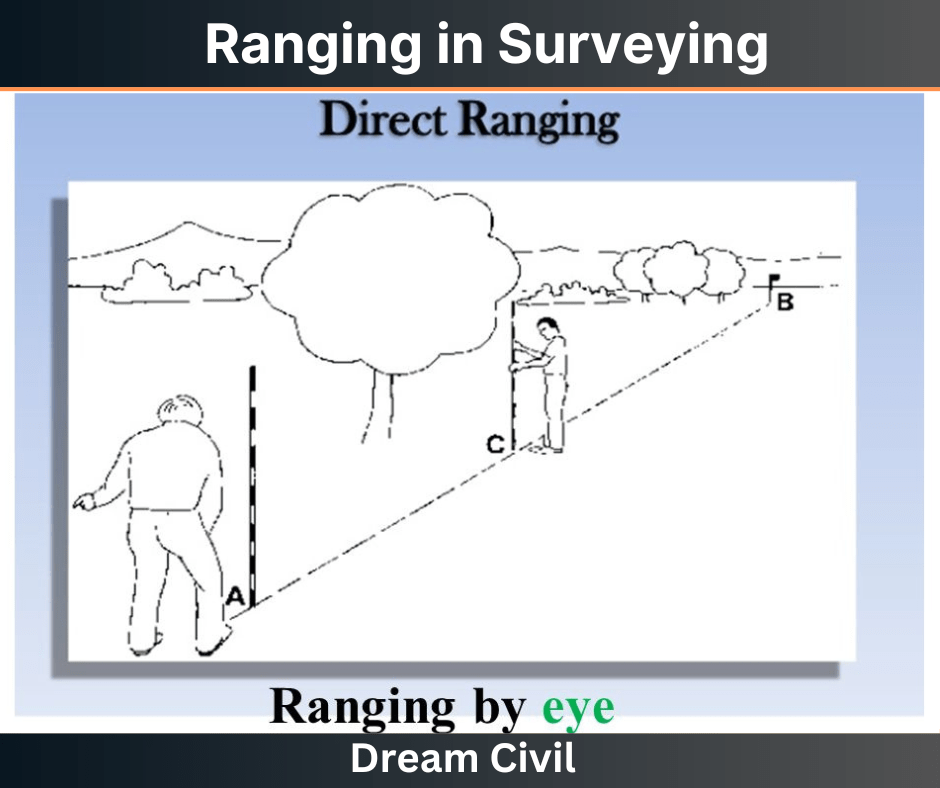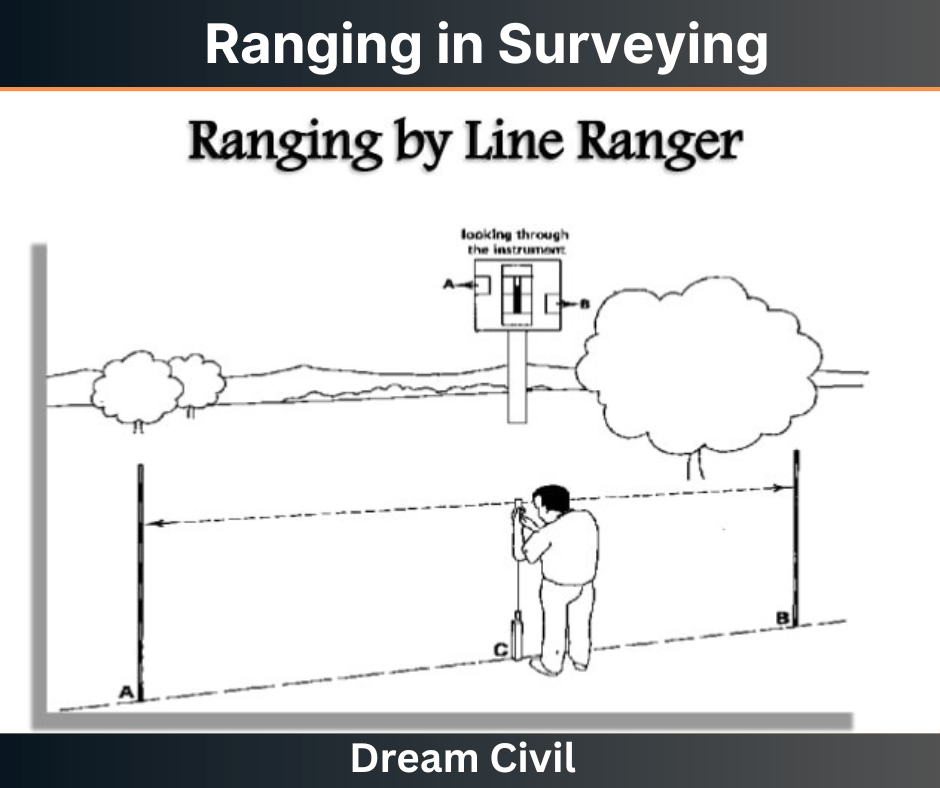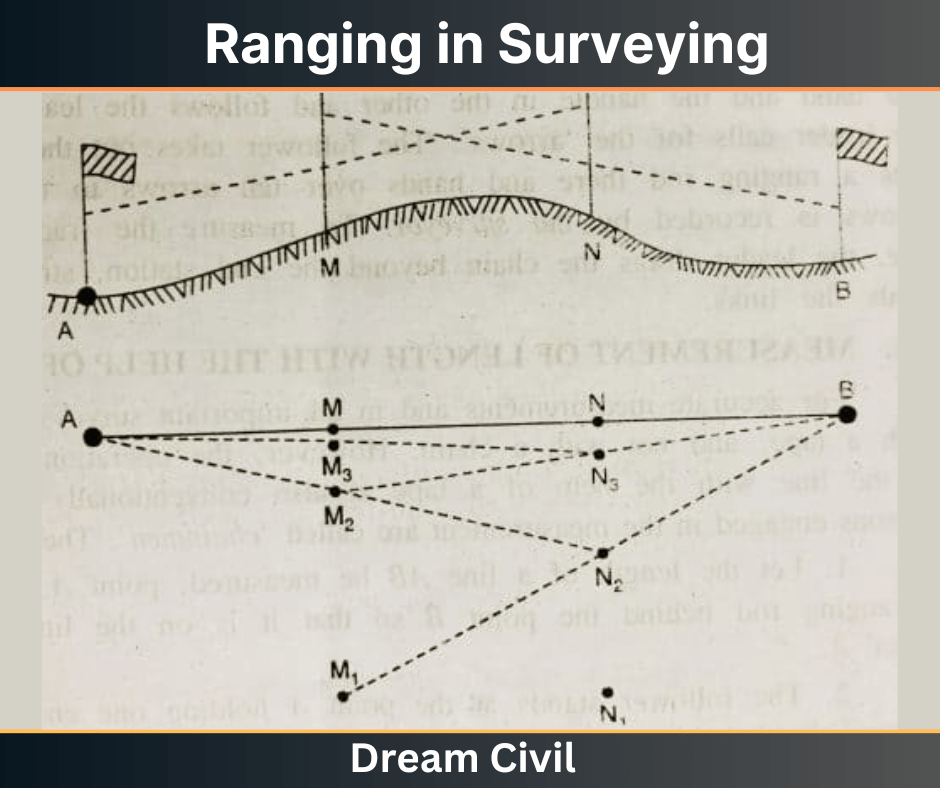Wednesday, October 4, 2023

# Ranging in Surveying | Types of Ranging | Direct Ranging | Indirect Ranging

The first step of any construction work is surveying and thus it is an important branch of civil engineering. In general, surveying can be defined as the art of determining the relative position of various features above, on, or beneath the surface of the earth utilizing different instruments and preparing maps. One of the important aspects of surveying is ranging in surveying.

In general, ranging can be defined as the process of fixing or establishing several intermediate points to measure the survey line.

The establishment of fixation of such intermediate points is accomplished utilizing ranging rods or ranging poles.

Generally, when the length of the survey line is longer than the length of the chain, the total length cannot be determined by chaining alone. In such a case, the intermediate points along the survey line are determined by ranging.(In other words:

In case, the length of the survey line is lesser than the length of the chain, the length of the line can easily be determined but if the length of the survey line is greater, then the survey lines have to be divided into certain intermediate points, before conducting the chaining process.

This process is known as ranging. There are two types of ranging in surveying that is commonly adopted i.e. direct ranging and indirect ranging.)

### 2. Types of Ranging in Surveying

The process of ranging in surveying can be categorized into two main types i.e. direct ranging and indirect ranging.

The types of ranging are further described below.

#### a. Direct Ranging

Direct ranging is the method of ranging that is adopted when the two survey stations are inter-visible i.e. the two endpoints of the survey line are visible.

In general, direct ranging can be further grouped into the following:

i. Ranging by Eye

It is the type of method of direct ranging that is done simply by the aid of the eyes of the surveyor.

As shown in the figure below, let A and B be the two inter-visible points at the ends of the survey line.

The surveyor then stands with a ranging rod at point A concerning point B.

Then, another person takes the ranging rod and fixes or establishes an intermediate point between the line AB. Let the intermediate point established be C.

It must be noted that the distance of the intermediate point is not greater than one chain length from the first point A of the survey line.

The surveyor at point A then signals another person at the intermediate points so that the ranging rod is in perfect line with the endpoints A and B. Hence, the intermediate points are determined.ii. Ranging by Line Ranger

Ranging by Line Ranger is the type of direct ranging method in which the intermediate points are fixed directly utilizing an instrument known as line ranger.

The line ranger is an instrument that has either two plane mirror arrangements or two isosceles prisms that are placed one over the other.

The arrangement and silvering of the diagonals of the prism are done such that they reflect incident rays.

Let us consider two endpoints of a survey line namely A and B as shown in the figure below.

At first, the surveyor places two rods at points A and B.

Let C be the intermediate point to be fixed. The surveyor then holds the line ranger at intermediate point C simply by eye judgment.

Then, the lower prism of the ranger receives the rays coming from point A. This ray is reflected by the diagonal towards the observer.

Similarly, the upper prism of the ranger receives the rays coming from point B. This ray is then reflected by the diagonal towards the observer.

Thus, the observer can sight both the images of the ranging rods at points A and B.

The images of the ranging rods are not in the same vertical line initially. Hence, the surveyor then moves the line ranger till the two images of the ranging rods lie in the same vertical line.

Once the images are in the same line, point C is transferred to the ground utilizing the plumb bob.

The major advantage of this method over the eye judgment method is that this method can be conducted easily by one person. The accuracy achieved by this method is also higher.#### b. Indirect Ranging

Indirect Ranging is the method of ranging that is used when the two endpoints of the survey line are either not inter-visible or the two points are at a very long distance.

As shown in the figure below, first of all, the two intermediate points M1 and N1 are located close to the chain such that from point M1 both N1 and B are visible.

Similarly from N1 both M1 and A must be visible.

Then, two ranging rods are placed at the points M1 and N1. Then, the surveyor at M1 signals the surveyor at N1 to move to the new point N2.

It must be noted that the new point N2 must be in line with the line M1B. Likewise, the surveyor at point N2 signals the surveyor at M1 to move to position M2.

It must be noted that the new point M2 is in line with line N2A. Finally, the two surveyors are at points M2 and N2.

This process is then repeated continuously until the points M and N are in line with the actual survey line AB.

After the final points, M and N are fixed, other successive points are easily fixed by direct ranging.### 3. Sources of Errors in Ranging in Surveying

a. Improper adjustment of ranging rods between two points of the survey line.

b. Inaccurate readings in hilly regions due to too many undulations.

### 4. References

1. Content Filter & Authenticity Checking Team, Dream Civil International

(Our team checks every content & detail to maintain quality.)He is a founder and lead author of Dream Civil International and his civil engineering research articles has been taken as source by world's top news and educational sites like USA Today, Time, The richest, Wikipedia, etc.
Latest Articles

Related Articles